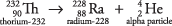If you look at the periodic table, you will notice that all elements after bismuth, atomic number 83, have their atomic mass denoted by an integer within parentheses. Such large nuclei are unstable and undergo radioactivity, the spontaneous disintegration by the emission of particles. This process is also known as radioactive decay. The atomic mass shown on the periodic table is the mass number of the most common isotope of each radioactive element.

For chemical purposes, the most important types of radiation are alpha and beta particles. An alpha particle (α) is anucleus with 2 protons and 2 neutrons. This nuclear notation uses a subscript to the lower left to record the number of protons, whereas the superscript to the upper left is the mass number, the total number of nucleons. The number of protons identifies the chemical element, whereas the nucleon total is the mass number for the particle or isotope.

If an unstable nucleus emits an alpha particle, its atomic number decreases by 2 and its mass number decreases by 4. The decay of thorium‐232 provides an example:Notice that radioactive decay actually changes one chemical element to another element, a process referred to as transmutation. Also notice that the total of the mass numbers on each side are the same, indicating they are conserved. This is also true for the total charge on each side.

The other chemically important mode of radioactive transmutation is provided by negative beta particles (β), which are electrons emitted by atomic nuclei, not from the surrounding electronic orbitals. The beta particle can be denoted as an electron (e ) or with the Greek letter beta (). The beta particle arises from the decay of a neutron to a proton:The creation of the proton causes the atomic number to increase by one. An example of beta transmutation is the decay of lead‐212:The atomic number increased to 83 due to the new proton, but the mass number stayed constant because both metal nuclei have 212 total protons plus neutrons.

The most familiar radioactive element is uranium, which has two naturally occurring isotopes of mass numbers 235 and 238 that decay very slowly. Review the first few steps in the decay of uranium‐238, which changes to lead‐206 after the emission of eight alpha and six beta particles. The earliest stages of the decay scheme involve only three elements, as shown in Figure 1.